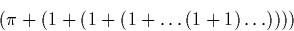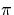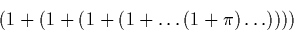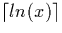Next: Summary of Theoretical Analysis Up: Theoretical Analysis Previous: Signed Binary Arithmetic

## Sequences of operations

One interesting consideration is the following. Suppose we wish to computeUsing signed binary (mantissa, exponent) representation we would require two digits ofto obtain one digit of output, although many more digits of the later numerals representing the number one. Suppose on the other hand we compute:Suppose we had 100 additions, we may require 101 digits from, although the result of the computation is the same. This implies that applying the operations sensibly, and computing expressions in a sort of tree form would be sensible. For example, although both results are the same,

(((a+a)+(a+a)) + ((a+a)+(a+a))) + (((a+a)+(a+a)) + ((a+a)+(a+a)))

requires less digits of a than

(a+(a+(a+(a+(a+(a+(a+(a+(a+(a+(a+(a+(a+(a+(a+a)))))))))))))))

The first example requires only four digits of a to obtain one digit of output, whereas the second requires sixteen. In general if the second form requires n digits of a, one would expect the first form to requiredigits.

Martin Escardo
5/11/2000